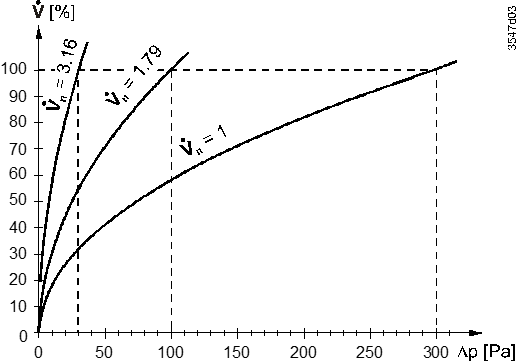# Measuring principle - Basic Documentation - GDB181.1E/BA - GDB181.1EMBA - GLB181.1E/BA

## VAV Compact Controller BACnet MS/TP G..B181.1E/BA

Product Family
OpenAir
Product Group
Damper actuators
Content Language
English
Content Type
Basic Documentation
Product Version
Status
Valid
Date
2021-10-26
Document No
A6V10631864_en--_g
A6V10695831
Stock No.
S55499-D168
S55499-D169
S55499-D506
Product No.
GDB181.1E/BA
GDB181.1EMBA
GLB181.1E/BA

A measuring device for acquiring the differential pressure – usually a measuring cross, measuring orifice or Venturi tube in the airflow – represents the basis for air volume flow measurement.

Differential pressure sensor

The air volume flow is measured indirectly with a differential pressure sensor. Since the measured value is the differential pressure Δp, the air flow is derived from this value using the VAV box characteristic. Accordingly, the VAV box OEM has to provide the combination of Δpnom and Vnom, out of which the air volume flow in m3/h or l/s can be calculated.

The air volume flow value (relative or absolute) can be transmitted over the bus together with the actual value of the damper position (in %) to be used by a supervisory controller or for management purposes. The differential pressure sensor operates long-term stable and without recalibration.

Note

In critical cases material compatibility tests should be made while considering harmful substances and concentrations.

Setting the characteristic value Vn

The parameter Vn is used to adjust the operating range of the differential pressure sensor (0…300 Pa) to the actual VAV box nominal pressure Δpnom at the factory. The effect of Vn is illustrated in the diagram below.

Effect of VnCalculation of Vn

(Δpnom = nominal differential pressure)

$$V_{n} = \sqrt{\frac{300\ \lbrack Pa\rbrack}{\mathrm{\Delta}p_{\text{nom}}\lbrack Pa\rbrack\ }}$$

Calculation example

Assume that a VAV box is designed for a nominal pressure of Δpnom = 120 Pa. Then Vn must be set to 1.58:

$$V_{n} = \sqrt{\frac{300\ Pa}{120\ Pa\ }} = 1.58$$